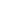FLAT 50% OFF

ENDS IN

• JEE Main
•• Mathematics
• Back to Doubt Clearing

## If $\sqrt{x+y}+\sqrt{y-x}=c$, then $\frac{d^{2} y}{d x^{2}}$ equals (a) $2 / c$(b) $-2 / c^{2}$(c) $2/ c^{2}$(d) None

### Asked By Pavan Dharimireddy

Updated Fri, 07 Jun 2019 12:05 pm